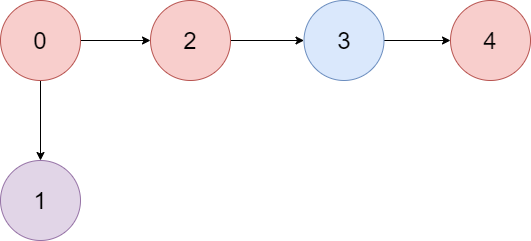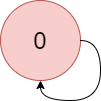1857. Largest Color Value in a Directed Graph
Hard
1.8K
61

There is a directed graph of `n` colored nodes and `m` edges. The nodes are numbered from `0` to `n - 1`.

You are given a string `colors` where `colors[i]` is a lowercase English letter representing the color of the `ith` node in this graph (0-indexed). You are also given a 2D array `edges` where `edges[j] = [aj, bj]` indicates that there is a directed edge from node `aj` to node `bj`.

A valid path in the graph is a sequence of nodes `x1 -> x2 -> x3 -> ... -> xk` such that there is a directed edge from `xi` to `xi+1` for every `1 <= i < k`. The color value of the path is the number of nodes that are colored the most frequently occurring color along that path.

Return the largest color value of any valid path in the given graph, or `-1` if the graph contains a cycle.

Example 1:```Input: colors = "abaca", edges = [[0,1],[0,2],[2,3],[3,4]]
Output: 3
Explanation: The path 0 -> 2 -> 3 -> 4 contains 3 nodes that are colored `"a" (red in the above image)`.
```

Example 2:```Input: colors = "a", edges = [[0,0]]
Output: -1
Explanation: There is a cycle from 0 to 0.
```

Constraints:

• `n == colors.length`
• `m == edges.length`
• `1 <= n <= 105`
• `0 <= m <= 105`
• `colors` consists of lowercase English letters.
• `0 <= aj, bj < n`
Accepted
55.4K
Submissions
106.7K
Acceptance Rate
51.9%

Seen this question in a real interview before?
1/4
Yes
No

Discussion (0)

Related Topics